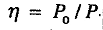# Effort Required to Move the Body on an Inclined Plane

Effort Required to Move the Body Up an Inclined Plane

The effort (P) required to move the body up an inclined plane as shown in Fig. 1.20, is given by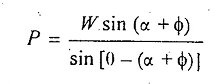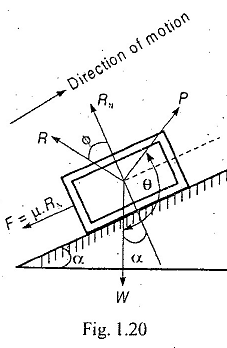where
W = Weight of the body,
α = Angle of inclination of the plane with the horizontal,
θ = Angle which the line of action of P makes with the weight of the body W, and
ϕ = Angle of friction.

Notes :

1. When friction is neglected, then ϕ = 0, In that case,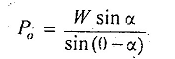2. When effort P is applied horizontally, then θ = 90°. In that case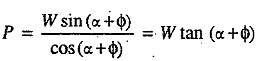3. When effort P is applied parallel to the plane, then θ = 90° + α. In that case,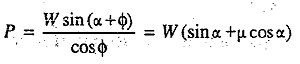Effort Required to Move the Body Down an Inclined Plane

The effort (P) required to Move the body down an inclined plane as shown in Fig. 1.21, is given by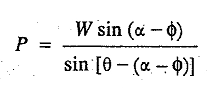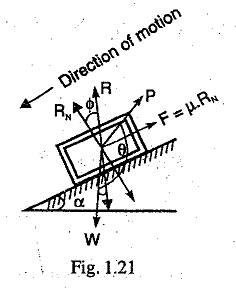Notes:
1. When friction is neglected, then ϕ = 0. In that case,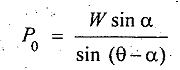2. When effort is applied horizontally, then θ = 90°. In That case,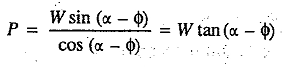3. When effort is applied parallel to the plane, then θ = 90° + α. In that case,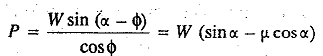Efficiency of an Inclined Plane

It is defined as the ratio of the effort required neglecting friction ( i.e. Po) to the effort required considering friction (i.e. P). Mathematically, efficiency of an inclined plane,